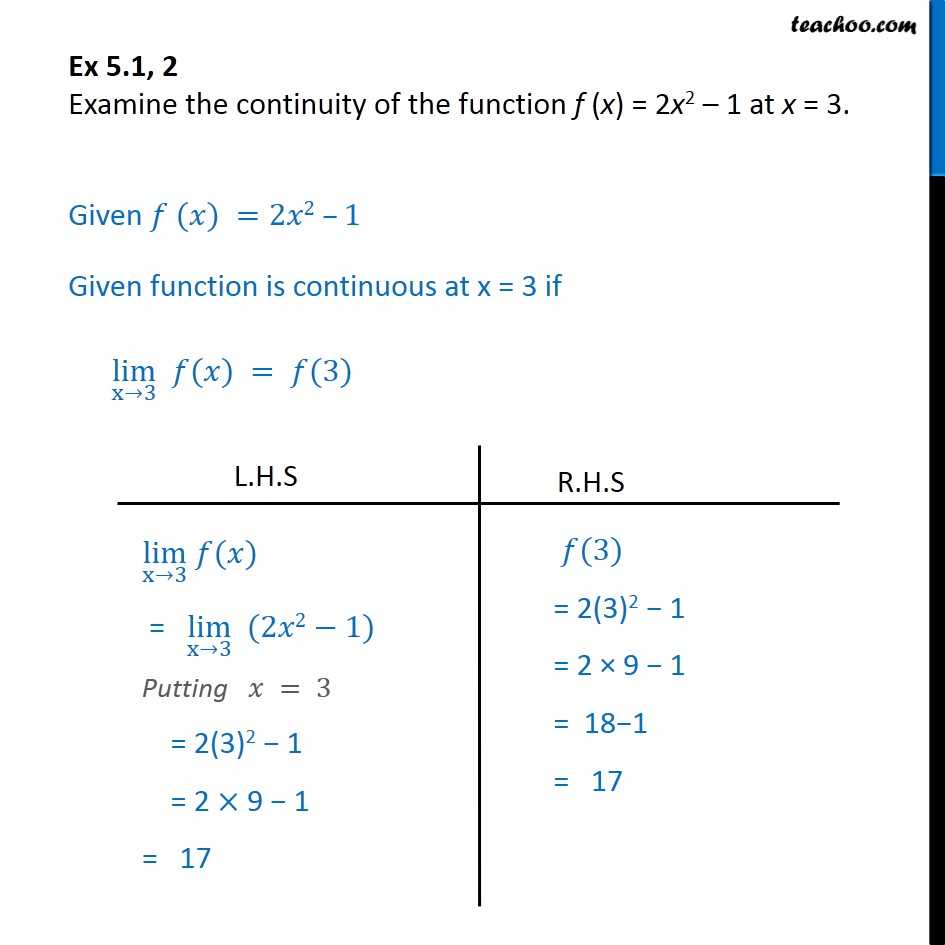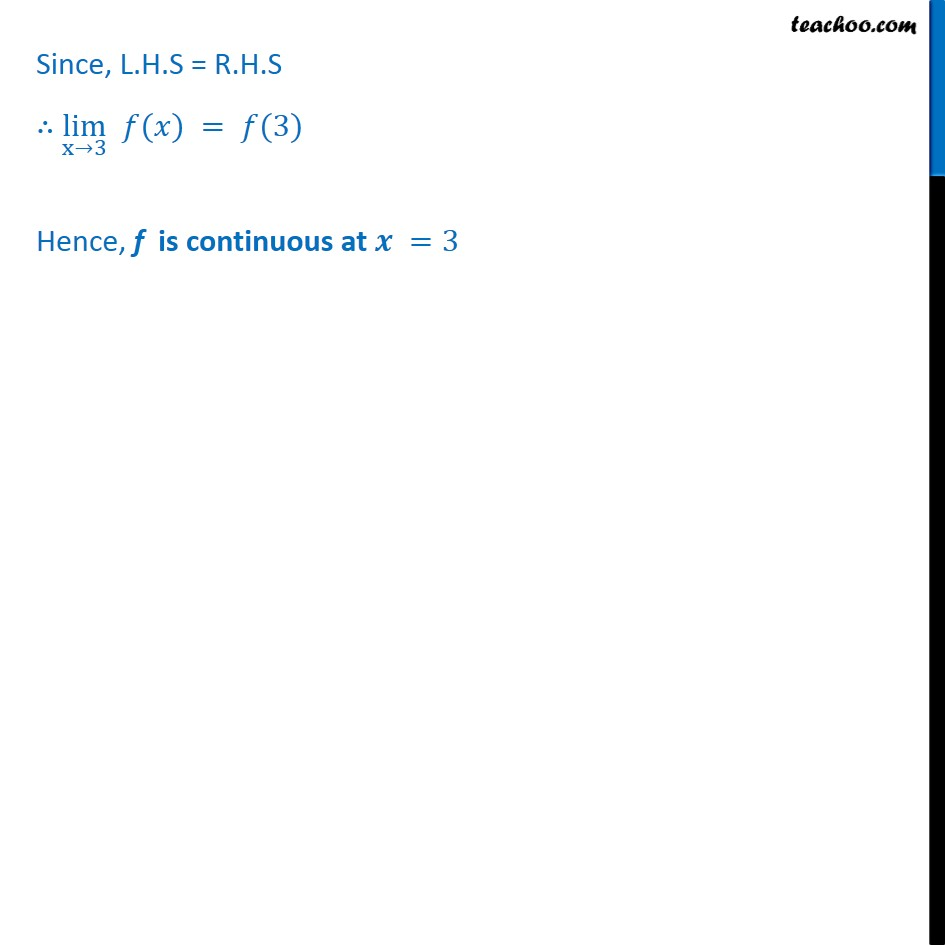Check Full Chapter Explained - Continuity and Differentiability - Continuity and Differentiability Class 121. Chapter 5 Class 12 Continuity and Differentiability
2. Serial order wise
3. Ex 5.1

Transcript

Ex 5.1, 2 - Chapter 5 Class 12 Continuity and Differentiability - NCERT Examine the continuity of the function f (x) = 2x^2 - 1 at x = 3. Given f(x) = 2x^2 - 1 Given function is continuous at x = 3 if lim x->3 f(x) = f(3) LHS lim x->3 f(x) = lim x->3 (2x^2 - 1) Putting x = 3 = 2 (3)^2 - 1 = 2 (9) - 1 = 18 - 1 = 17 RHS f(3) = 2 (3)^2 - 1 = 2 (9) - 1 = 18 - 1 = 17 Since, L.H.S = R.H.S lim x->3 f(x) = f(3) Hence, f(x) is continuous at x = 3

Ex 5.1